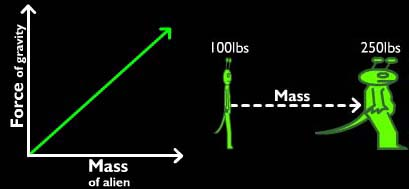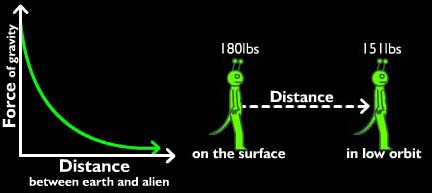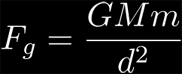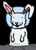### Gravitation

##### Gravity and MassFrom the graph we can see that as the mass of the alien increases, the gravitational force (the alien's weight) also increases: mass varies directly with gravitational force. The more massive two objects are, the greater the gravitational attraction between them.

##### Forceg ∝ Mass

Click the "Increase Mass" button to see the effect of increased mass on gravitational attraction.

##### Gravity and DistanceThe force of gravity is inversely related to the distance the alien is from Earth; this means that force of gravity decreases as the distance between the alien and Earth increases.

As the distance between the alien and the surface of the planet increases, the force of gravity decreases. This relationship is true in all cases: as the distance increases between any two objects, the gravitational force gets much smaller very fast in an inverse square relationship.

##### Forceg ∝ 1/d2

As distance increases, gravitational attraction decreases.

##### Putting it Together: The Universal Law of Gravitation

Newton combined the inverse square relation between distance and gravitational attraction with the direct relation between mass and gravitational attraction as well as an additional constant of proportionality. His end result would be one of the most powerful laws in classical physics: the Universal Law of Gravitation.Where:

Fg = force between objects 1 and 2
G (constant of proportionality) = 6.67 x 10-11 Nm2/kg2 (from experiments)
M = mass of first object
m = mass of second object
d = distance between the center of each object[1.9a] Down the Rabbit Hole: Mass and Weight